# HSSlive: Plus One & Plus Two Notes & Solutions for Kerala State Board

## AP Board Class 7 Maths Chapter 9 Algebraic Expressions InText Questions Textbook Solutions PDF: Download Andhra Pradesh Board STD 7th Maths Chapter 9 Algebraic Expressions InText Questions Book AnswersAP Board Class 7 Maths Chapter 9 Algebraic Expressions InText Questions Textbook Solutions PDF: Download Andhra Pradesh Board STD 7th Maths Chapter 9 Algebraic Expressions InText Questions Book Answers

## Andhra Pradesh State Board Class 7th Maths Chapter 9 Algebraic Expressions InText Questions Books Solutions

 Board AP Board Materials Textbook Solutions/Guide Format DOC/PDF Class 7th Subject Maths Chapters Maths Chapter 9 Algebraic Expressions InText Questions Provider Hsslive

2. Click on the Andhra Pradesh Board Class 7th Maths Chapter 9 Algebraic Expressions InText Questions Answers.
3. Look for your Andhra Pradesh Board STD 7th Maths Chapter 9 Algebraic Expressions InText Questions Textbooks PDF.
4. Now download or read the Andhra Pradesh Board Class 7th Maths Chapter 9 Algebraic Expressions InText Questions Textbook Solutions for PDF Free.

## AP Board Class 7th Maths Chapter 9 Algebraic Expressions InText Questions Textbooks Solutions with Answer PDF Download

Find below the list of all AP Board Class 7th Maths Chapter 9 Algebraic Expressions InText Questions Textbook Solutions for PDF’s for you to download and prepare for the upcoming exams:

Question 1.
How many number of terms are there in each of the following expressions ?
(i) 5x2 + 3y + 7
Given expression is 5x2 + 3y + 7 Number of terms = 3

(ii) 5x2y + 3
Given expression is 5x2y + 3
Number of terms = 2

(iii) 3x2y
Given expression is 3x2y
Number of terms = 1

(iv) 5x – 7
Given expression is 5x – 7
Number of terms = 2

(v) 7x3 – 2x
Given expression is 7x3 – 2x
Number of terms = 2

Question 2.
Write numeric and algebraic terms in ‘ the above expressions.
(i) 5x2 + 3y + 7
Given expression is 5x2 + 3y + 7
Numerical terms = 7
Algebraic terms = 5x2, 3y

(ii) 5x2y + 3
Given expression is 5x2y + 3
Numerical terms = 3
Algebraic terms = 5x2y

(iii) 3x2y
Given expression is 3x2y
Numerical terms = No
Algebraic terms = 3x2y

(iv) 5x – 7
Given expression is 5x – 7
Numerical terms = – 7
Algebraic terms = 5x

(v) 7x3 – 2x
Given expression is 7x3 – 2x
Numerical terms = No
Algebraic terms = 7x3, – 2x

Question 3.
Write the terms in the following expressions.
– 3x + 4, 2x – 3y, 43a2 + 52b,
1.2ab + 5.1b – 3.2a

 Expressions Terms – 3x+4 – 3x, 4 2x – 3y 2x, – 3y 43a2 + 52b 43a2 + 52b 1.2 ab + 5.1 b – 3.2 a 1.2 ab, 5.1 b, – 3.2a

Let’s Explore (Page No: 50)

Question 1.
Identify the terms which contain m2 and write the coefficients of m2.
(i) mn2 + m2n
Given expression is mn2 + m2n

 m2 term Coefficeint m2n n

(ii) 7m2 – 5m – 3
Given expression is 7m2 – 5m – 3

 m2 term Coefficeint 7m2 7

(iii) 11 – 5m2 + n + 8 mn
Given expression is 11 – 5m2 + n + 8 mn

 m2 term Coefficeint – 5m2 -5

Check Your Progress [Page No. 52]

Question 1.
Write like terms from the following :
– xy2, – 4yx, 8x, 2xy2, 7y, – 11x2, – 100x, – 11yx, 20x2y, – 6x2, y, 2xy, 3x
Given – xy2, – 4yx, 8x, 2xy2, 7y, – 11x2, – 100x, – 11yx, 20x2y, – 6x2, y, 2xy, 3x

• – xy2, 2xy2 are like terms because they contain same algebraic factor xy2.
• – 4yx, – 11yx, 2xy are like terms because they contain same algebraic factor xy.
• 8x, – 100x, 3x are like terms because they contain same algebraic factor x.
• 7y, y are like terms because they contain same algebraic factor y.
• – 11x2, – 6x2. are like terms because they contain same algebraic factor x2.

Question 2.
Write 3 like terms for
(i) 3x2y
Like terms of 3x2y are – 8x2y, 53 x2y, 2.9 x2y

(ii) – ab2c
Like terms of – ab2c are 3ab2c, 5.8 ab2c, 14 ab2c.

Let’s Explore [page No: 52]

Question 1.
Jasmin says that 3xyz is a trinomial. Is she right ? Give reason.
Given expression 3xyz.
In this expression only one term is there. So, it is a monomial only. But, not trinomial.
So, Jasmin is wrong.

Question 2.
Give two examples each for Monomial and Binomial algebraic expression.

 Type of Expressions Expressions Monomial x, b2c, xy2z, ……………….. Binomial x + 2y, 4b – 3c, x2y – yz, ……………

Check Your progress [Page No. 54]

Question 1.
(i) 12ab, 9ab, ab
Sum of 12ab, 9ab, ab
= 12ab + 9ab + ab
= (12 + 9 + 1) ab
= 22 ab.

(ii) 10x2, – 3x2, 5x2
Sum of 10x2, – 3x2, 5x2
= 10x2 + (- 3x2) + 5x2
= 10x2 – 3x2 + 5x2
= (10 – 3 + 5) x2
= 12x2

(iii) – y2, 5y2, 8y2, – 14y2
Sum of – y2, 5y2, 8y2, – 14y2
= – y2 + 5y2 + 8y2 + (- 14y2)
= – 1y2 + 5y2 + 8y2 – 14y2
= (- 1 + 5 + 8 – 14) y2
= – 2y2

(iv) 10mn, 6mn, – 2mn, – 7mn
Sum of 10mn, 6mn, – 2mn, – 7mn
= 10mn + 6mn + (- 2mn) + (- 7mn)
= 10mn + 6mn – 2mn – 7mn
= (10 + 6 – 2 – 7) mn
= 7mn

Let’s Think [Page No: 54]

Reshma simplified the expression
4p + 6p + p like this.
4p + 6p + p = 10p. Is she correct ?
4p + 6p + p = (4 + 6 + 1)p
= 11p ≠ 10p
So, Reshma simplified is wrong.

Check Your Progress [Page No: 54]

Question 1.
Write the standard form of the following expressions :
(i) – 5l + 2l2 + 4
2l2 – 5l + A

(ii) 4b2 + 5 – 3b
4b2 – 3b + 5

(iii) z – y – x
– x – y + z.

Check Your Progress [Page No. 56]

Question 1.
Add the following expressions in both Row and Column methods:
(i) x – 2y, 3x + 4y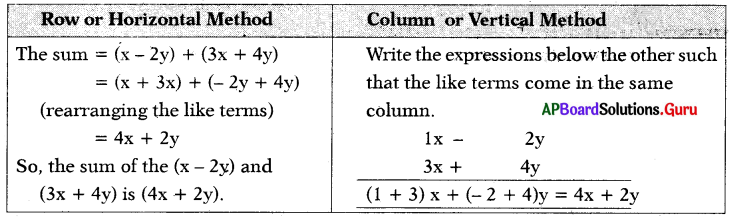(ii) 4m2 – 7n2 + 5mn, 3n2 + 5m2 – 2mn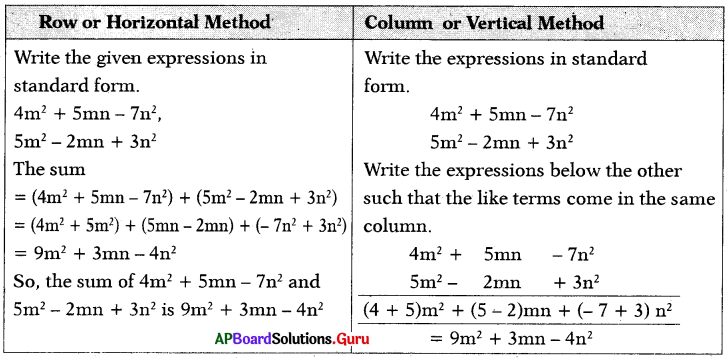(iii) 3a – 4b, 5c – 7a + 2b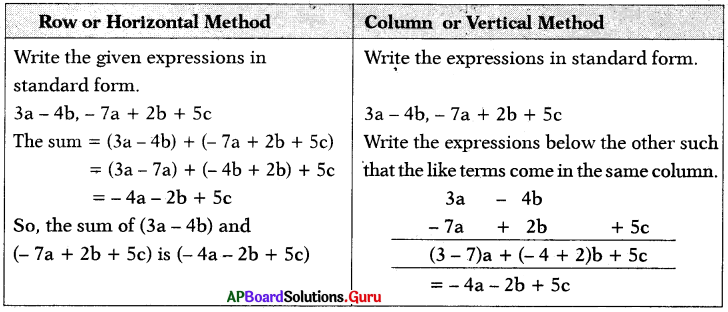Let’s Explore [Page No. 56]

Think of at least two situations in each of which you need to form two algebraic expressions and add them.

(i) Aditya and Madhu went to a store. Aditya bought 6 books and Madhu bought 2 books. All the books are same cost. How much money did both spend ₹
Let the cost of each book ₹a.
Number of books Aditya bought = 6
Cost of 6 books = 6 × a = ₹ 6a
Number of books Madhu bought = 2
Cost of 2 books = 2 × a = ₹ 2a
= 6a + 2a
= (6 + 2)a
= ₹ 8a

(ii) Sreeja and Sreekari went to icecream parlour. Sreeja bought Vineela icecreams 2 and Sreekari bought Butter Scotch 3. Cost of icecreams are different. How much money they gave to the shop keeper ₹
Let the cost of Vineela is ₹x and cost of Butter Scotch is ₹y.
Number of Vineela icecreams Sreeja bought = 2
Cost of 2 iceqreams = 2 × x = ₹ 2x
Number of Butter Scotch icecreams Sreekari bought = 3
Cost of 3 icecreams = 3 × y = ₹ 3y
Money given to shopkeeper = 2x + 3y
= ₹(2x + 3y)

Check Your Progress [Page No. 57]

Question 1.
Subtract the first term from second term :
(i) 2xy, 7xy
7xy – 2xy
= (7 – 2) xy
= 5xy

(ii) 4a2, 10a2
10a2 – 4a2 = (10 – 4)a2 = 6a2

(iii) 15p, 3p
3p – 15p = (3 – 15)p = – 12p

(iv) 6m2n, – 20m2n
– 20 m2n – 6m2n = (- 20 – 6) m2n
= – 26m2n

(v) a2b2, – a2b2
– a2b2 – a2b2 = (- 1 – 1)a2b2 = – 2a2b2

Let’s Explore [Page No. 58]

Add and subtract the following expressions in both Horizontal and Vertical method x – 4y + z, 6z – 2x + 2y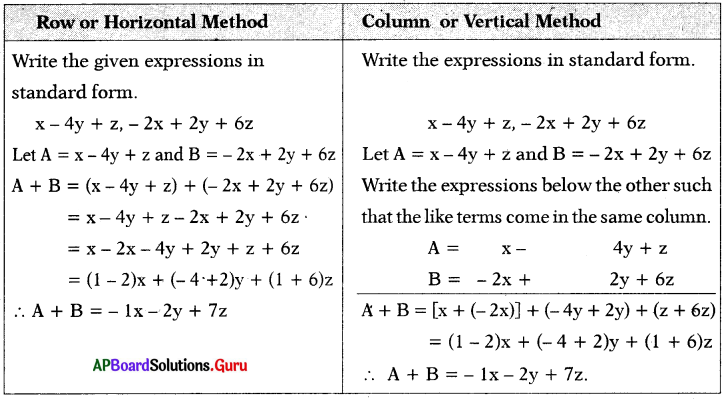Subtraction: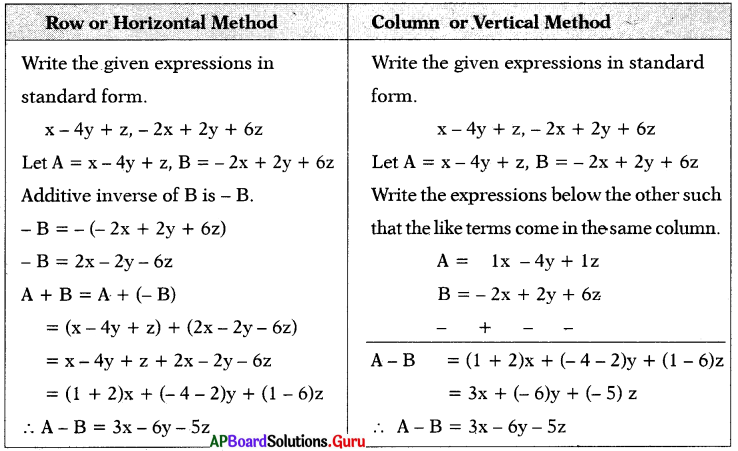Let’s Explore [Page No. 60]

Question 1.
Write an expression whose value is – 15 when x = – 5.
Given x = – 5 and value = – 15
Value = – 15 ,
= 3 × – 5 ,
= – 3 × x (∵ x = – 5)
∴ Expression = 3x

Question 2.
Write an expression whose value is 15 when x = 2.
Given x = 2 and value = 15
Value = 15
302
12 × 15 × 2
12 × 15 × x (∵ x = 2)
∴ Expression = 15𝑥2

Lets Think [Page No. 60]

While finding the value of an algebraic expression 5x when x = – 2, two stu¬dents solved as follows: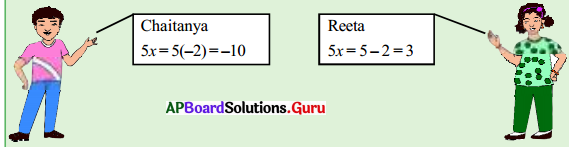Can you guess who has done it correctly? Justify!
Given expression is 5x when x = – 2
5x = 5(- 2) = – 10
Chaitanya is correct.
Here we have to multiply 5 and,- 2.
But Reeta subtracted.
So, Reeta is wrong.

Examples

Question 1.
How many number of terms are there in each of the following expressions ?
(i) a + b
a + b ……….. 2 terms

(ii) 3t2
3t2 ……….. 1 term

(iii) 9p3 + 10q – 15
9p3 + 10q – 15 ……….. 3 terms

(iv) 5 m3n
5 m3n ……….. 1 term

(v) 4x + 5y – 3z – 1
4x + 5y – 3z – 1 ……….. 4 terms

Question 2.
In the following expressions, write the number of terms and identify numeri¬cal and algebraic expressions in them.
(i) 8p
8p = 1 term – Algebraic expression

(ii) 5c + s – 7
5c + s – 7 = 3 terms – Algebraic expression

(iii) – 6
– 6 = 1 term – Numerical expression

(iv) (2 + 1) – 6
(2 + 1) – 6 = 2 terms – Numerical expression

(v) 9t + 15
9t + 15 = 2 terms – Algebraic expression

Question 3.
Write the coefficients of
(i) p in 8pq
8pq = p(8q)
so, coefficient of p in 8pq is 8q.

(ii) x in 𝑥𝑦3
𝑥𝑦3 = x(y3)
so, coefficient of x in 𝑥𝑦3 is (y3)

(iii) abc in (- abc)
(- abc) = – (abc)
so, coefficient of abc is – 1.

Question 4.
Identify like terms among the following and group them:
10ab, 7a, 8b, – a2b2, – 7ba, – 105b, 9b2a2, – 5a2, 90a.
(7a, 90a) are like terms because they
contain same algebraic factor ‘a’.
(10ab, – 7ba) are like terms as they have same algebraic factor ’ab’.
(8b, -105b) are like terms because they contain same algebraic factor ‘b’.
(- a2b2, 9b2a2) are like terms because they contain same algebraic factor ‘a2b2‘.

Question 5.
State with reasons, classify the following expressions into monomials, binomials, trinomials.
a + 4b, 3x2y, px2 + qx + 2, qz2, x2 + 2y, 7xyz, 7x2 + 9y3 – 10z4, 3l2 – m2, x, – abc.

 Expressions Type of the Expression Reason x, 7xyz, 3x2y, qz2, – abc Monomial One term a + 4b, x2 + 2y, 3l2 – m2 Binomial Two unlike terms px2 + qx + 2, 7x2 + 9y3 – 10z4 Trinomial Three unlike terms

Question 6.
Find the sum of the following like terms :
(i) 3a, 9a
Sum of 3a, 9a = 3a + 9a
= (3 + 9)a = 12a

(ii) 5p2q, 2p2q
Sum of 5p2q, 2p2q = 5p2q + 2p2q
= (5 – 2) p2q = 7p2q

(iii) 6m, – 15m, 2m
Sum of 6m, – 15m, 2m
= 6m + (- 15m) + 2m
= 6m – 15m + 2m
= (6 – 15 + 2)m = – 7m

Question 7.
Write the perimeter of the given figure.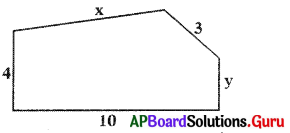The perimeter of the figure
= 10 + 4 + x + 3+ y
= x + y + (10 + 4 + 3)
= x + y + 17

Question 8.
Simplify:
6a2 + 3ab + 5b2 – 2ab – b2 + 2a2 + 4ab + 2b2 – a2
6a2 + 3ab + 5b2 – 2ab – b2 + 2a2 + 4ab + 2b2 – a2
= (6a2 + 2a2 – a2) + (3ab – 2ab + 4ab) + (5b2 – b2 + 2b2)
= [(6 + 2 – 1) a2] + [(3 – 2 + 4)ab] + [(5 – 1 + 2)b2]
= 7a2 + 5ab + 6b2

Question 9.
Add 2x2 – 3x + 5 and 9 + 6x2 in vertical method.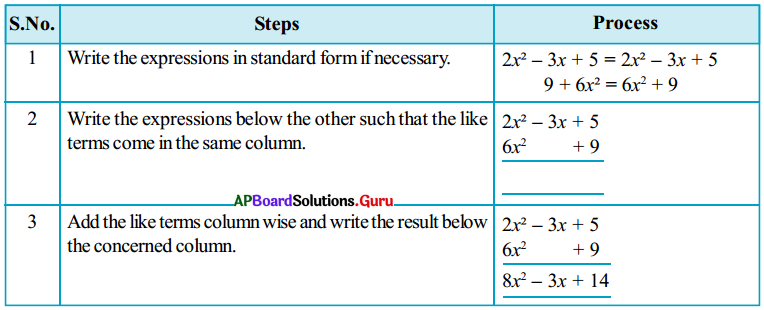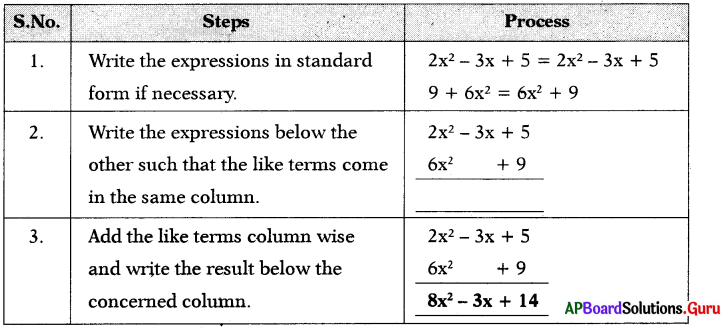Question 10.
Find the additive inverse of the follow-ing expressions:
(i) 35
Additive inverse of 35 = – 35

(ii) – 5a
Additive inverse of – 5a = – (-5a) = 5a

(iii) 3p – 7
Additive inverse of 3p – 7 = – (3p – 7) = – 3p + 7

(iv) 6x2 – 4x + 5
Additive inverse of 6x2 – 4x + 5
= (6x2 – 4x + 5) = – 6x2 + 4x – 5

Question 11.
Subtract 2p2 – 3 from 9p2 – 8.
9p2 – 8 – (2p2 – 3) = 9p2 – 8 – 2p2 + 3
= (9 – 2) p2 – 8 + 3
= 7p2 – 5

Question 12.
Subtract 3a + 4b – 2c from 6a – 2b + 3c in row method.
Let A = 6a – 2b + 3c, B = 3a + 4b – 2c
Subtracting 3a + 4b – 2c from 6a – 2b + 3c is equal to adding additive inverse of 3a + 4b – 2c to 6a – 2b + 3c
i.e., A – B = A + (-B)
additive inverse of (3a + 4b – 2c) = – (3a + 4b – 2c) = – 3a – 4b + 2c
A – B = A + (-B)
= 6a – 2b + 3c + (- 3a – 4b + 2c)
= 6a – 2b + 3c – 3a – 4b +2c = (6 – 3)a – (2 + 4)b + (3 + 2)c
Thus, the required answer = 3a – 6b + 5c

Question 13.
Subtract 3m3 + 4 from 6m3 + 4m2 + 7m – 3 in stepwise method.
Let us solve this in stepwise.
Step 1: 6m3 + 4m2 + 7m – 3 – (3m3 + 4)
Step 2: 6m3 + 4m2 + 7m – 3 – 3m3 – 4
Step 3: 6m3 – 3m3 + 4m2 + 7m – 3 – 4 (rearranging like terms)
Step 4: (6 – 3)m3 + 4m2 + 7m – 7 (distributive law)
Thus, the required answer = 3m3 + 4m2 + 7m – 7

Question 14.
Subtract 4m2 – 7n2 + 5mn from 3n2 + 5m2 – 2mn.
(For easy understanding, same colours are taken to like terms)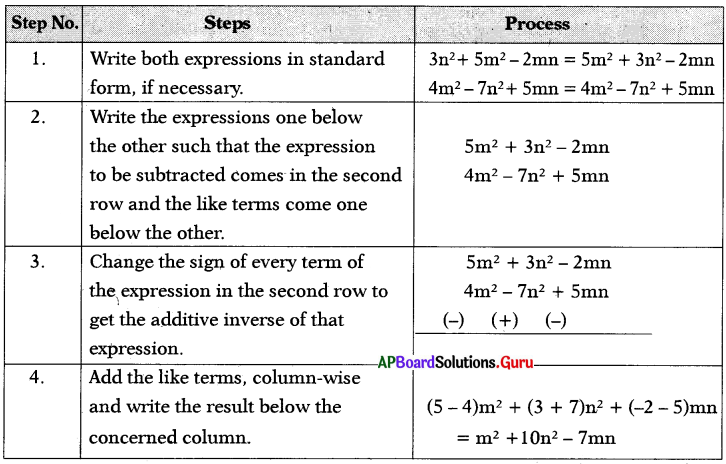Question 15.
Find the value of the following expressions, when x = 3.
(i) x + 6
When x = 3, the value of x + 6
(substituting 3 in the place of x) is
(3) + 6 = 9

(ii) 8x – 1
When x = 3,
the value of 8x – 1 = 8(3) – 1
= 24 – 1 = 23

(iii) 14 – 5x
When x = 3,
the value of 14 – 5x = 14 – 5(3)
= 14 – 15 = – 1

Practice Questions [Page No: 65]

Question 1.
In a certain code BOARD: CNBQE, how ANGLE will be written in that code?
(a) BMHKF
(b) CNIJE
(c) BLGIF
(d) CMIKF
(a) BMHKF

Explaination:
Each letter in a word is shifted to 1 position is alphabetical order and went 1 position is backward like this.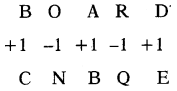ANGLE = BMHKF

Question 2.
In a certain code, MOBILE: 56, how PHONE will be written in that code?
(a) 52
(b) 54
(c) 56
(d) 58
(d) 58

Explaination:
Taking sum of positions of letters in for ward direction.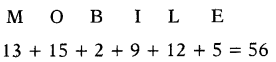So, PHONE = 16 + 8 + 15 + 14 + 5 = 58

Question 3.
If BEAN: ABNE, then NEWS ?
(a) WSNE
(b) WSEN
(c) WNSE
(d) WNES
(c) WNSE

Explaination:
Follow the same to decode the given word.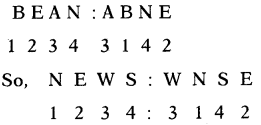Question 4.
If ROSE : 6821, CHAIR : 73456, PREACH : 961473, then SEARCH ?
(a) 241673
(b) 214673
(c) 216473
(d) 216743
(b) 214673

Explaination:
By comparing each letter with the symbol, by writing one below the other.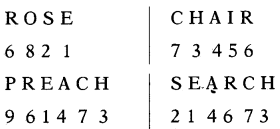Question 5.
If COMPUTER: RFUVQNPC, then MEDICINE?
(a) EDJOJMEF
(b) EOJDJEFM
(c) EOJJDFEM
(d) EDJJOFME
(b) EOJDJEFM

Explaination:
In the coded form the first & last letters have been interchanged while the rem¬aining letters are coded by taking their immediate next letters in the reverse order.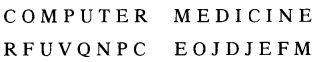Question 6.
If LAKE = 7@\$5, WALK = %@7\$, then WAKE = ?
(a) @%75
(b) %@\$5
(c) %5@7
(d) %@57
(b) %@\$5

Explaination:
By comparing each letter with symbol, by writing One below the other.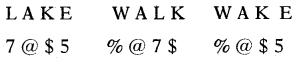Question 7.
If MANY = OCPA, then LOOK = ?
(a) NQQM
(b) MQQN
(c) QMQN
(d) QNQM
(a) NQQM

Explaination:
Each letter in a word is shifted to 2 position forward in alphabetical order.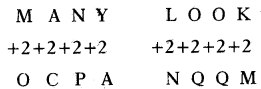∴ LOOK = NQQM

Question 8.
If SOME = PUB, then BODY ?‘
(a) LABY
(b)YBAL
(c) YLAV
(d) ABLY
(c) YLAV

Explaination:
Bach letter in a word is shifted to 3 position backward in alphabetical order.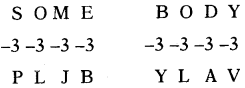So, BODY = YLAV

Question 9.
If ARC = CVI, then RAY?
(a) TEU
(b) TEE
(c) TED
(d) TEF
(b) TEE

Explaination:
Each letter in a word is forwarded alphabetical order as follows.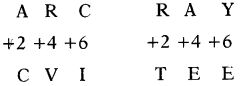So, RAY = TEE

Question 10.
If MEAN = KGYI, then MODE = ?
(a) QBGK
(b) KBQC
(c) KGBQ
(d) kQBG
(d) kQBG

Explaination:
Each letter in the word is shifted to 2 position backward and 2 position forward as following.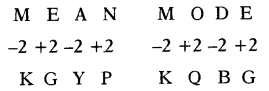So, MODE = KQBG

Question 11.
If FIND = DNIF, then DONE ?
(a) ENOD
(b) ENDO
(c) NEOD
(d) ONED
(a) ENOD

Explaination:
By reversing the word from left to right.
So, DONE = ENOD .

Question 12.
If BASE = SBEA, then AREA = ?
(a) AARE
(b) EAAR
(c) EARA
(d) REAA
(b) EAAR

Explaination:
Follow the same to decode the given word.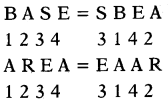Question 13.
If LESS = 55, then MORE = ?
(a)54
(b)50
(c) 51
(d) 52
(c) 51

Explaination:
Taking sum of positions of letters in forward direction.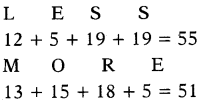Question 14.
If BACK = 17, then CELL =?
(a) 33
(b) 30
(c) 31
(d) 32
(d) 32

Explaination:
Taking sum of positions of letters in for ward direction.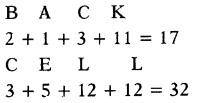3 + 5 + 12 + 12 = 32

Question 15.
If BIG = 63, then SMALL =?
(a) 76
(b) 78
(c) 74
(d) 72
(b) 78

Explaination:
Taking sum of positions of letters in reverse direction.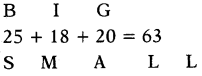8 + 14 + 26 + 15 + 15 = 78

## Andhra Pradesh Board Class 7th Maths Chapter 9 Algebraic Expressions InText Questions Textbooks for Exam Preparations

Andhra Pradesh Board Class 7th Maths Chapter 9 Algebraic Expressions InText Questions Textbook Solutions can be of great help in your Andhra Pradesh Board Class 7th Maths Chapter 9 Algebraic Expressions InText Questions exam preparation. The AP Board STD 7th Maths Chapter 9 Algebraic Expressions InText Questions Textbooks study material, used with the English medium textbooks, can help you complete the entire Class 7th Maths Chapter 9 Algebraic Expressions InText Questions Books State Board syllabus with maximum efficiency.

## FAQs Regarding Andhra Pradesh Board Class 7th Maths Chapter 9 Algebraic Expressions InText Questions Textbook Solutions

#### Can we get a Andhra Pradesh State Board Book PDF for all Classes?

Yes you can get Andhra Pradesh Board Text Book PDF for all classes using the links provided in the above article.

## Important Terms

Andhra Pradesh Board Class 7th Maths Chapter 9 Algebraic Expressions InText Questions, AP Board Class 7th Maths Chapter 9 Algebraic Expressions InText Questions Textbooks, Andhra Pradesh State Board Class 7th Maths Chapter 9 Algebraic Expressions InText Questions, Andhra Pradesh State Board Class 7th Maths Chapter 9 Algebraic Expressions InText Questions Textbook solutions, AP Board Class 7th Maths Chapter 9 Algebraic Expressions InText Questions Textbooks Solutions, Andhra Pradesh Board STD 7th Maths Chapter 9 Algebraic Expressions InText Questions, AP Board STD 7th Maths Chapter 9 Algebraic Expressions InText Questions Textbooks, Andhra Pradesh State Board STD 7th Maths Chapter 9 Algebraic Expressions InText Questions, Andhra Pradesh State Board STD 7th Maths Chapter 9 Algebraic Expressions InText Questions Textbook solutions, AP Board STD 7th Maths Chapter 9 Algebraic Expressions InText Questions Textbooks Solutions,
Share: